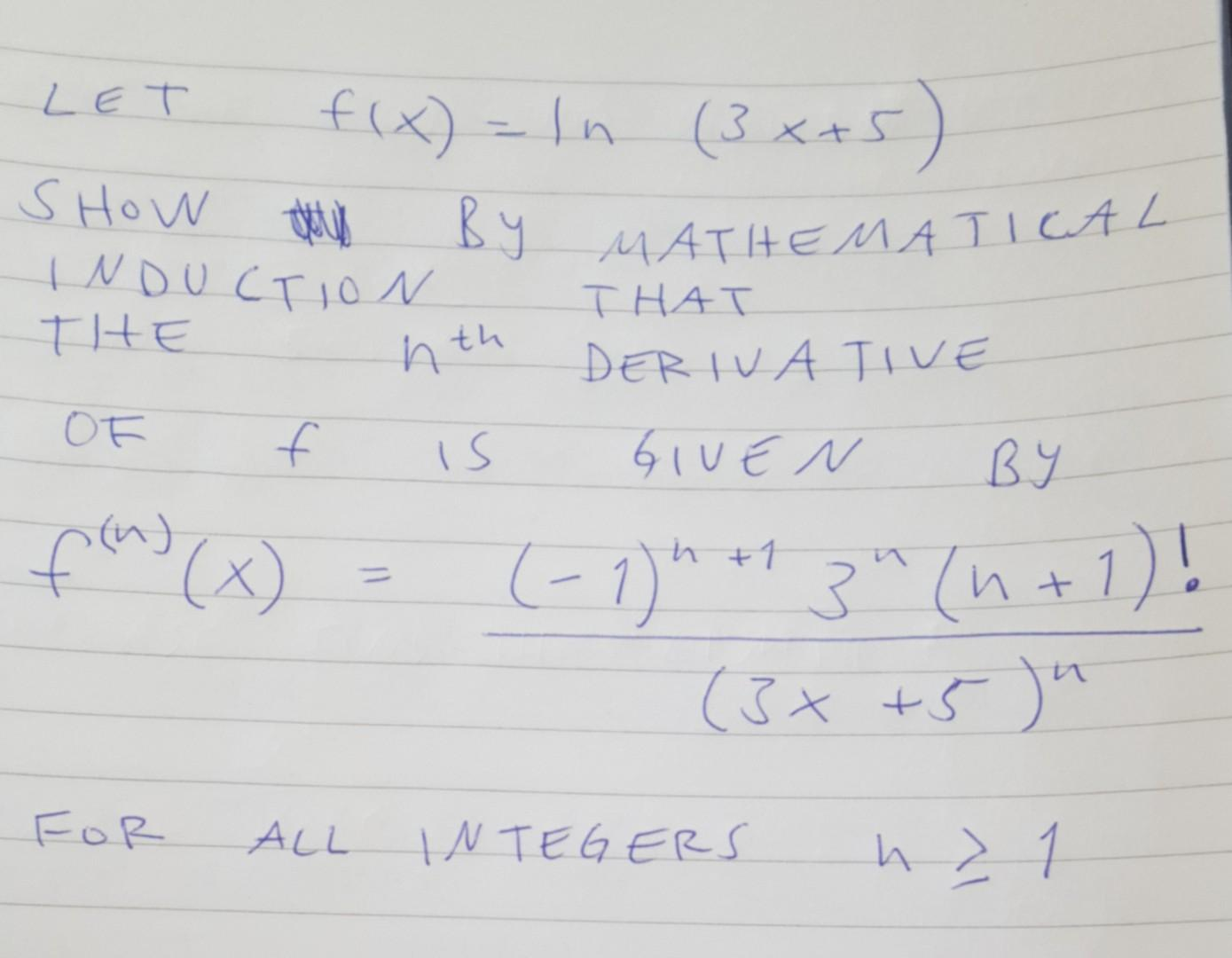# Question   $\text { LET } f(x)=\ln (3 x+5)$ SHOW BY MATHEMATICAL $\begin{array}{l} \text { INDUCTION MATIEMATH THT } \\ \text { THE } n^{\text {th }} \text { DERIVATIVE } \\ \text { OF } f \text { IS GIVEN BY } \\ f^{(n)}(x)=\frac{(-1)^{n+1} 3^{n}(n+1) !}{(3 x+5)^{n}} \end{array}$ For all INTEGERS $$h \geqslant 1$$Transcribed Image Text: $\text { LET } f(x)=\ln (3 x+5)$ SHOW BY MATHEMATICAL $\begin{array}{l} \text { INDUCTION MATIEMATH THT } \\ \text { THE } n^{\text {th }} \text { DERIVATIVE } \\ \text { OF } f \text { IS GIVEN BY } \\ f^{(n)}(x)=\frac{(-1)^{n+1} 3^{n}(n+1) !}{(3 x+5)^{n}} \end{array}$ For all INTEGERS $$h \geqslant 1$$
Transcribed Image Text: $\text { LET } f(x)=\ln (3 x+5)$ SHOW BY MATHEMATICAL $\begin{array}{l} \text { INDUCTION MATIEMATH THT } \\ \text { THE } n^{\text {th }} \text { DERIVATIVE } \\ \text { OF } f \text { IS GIVEN BY } \\ f^{(n)}(x)=\frac{(-1)^{n+1} 3^{n}(n+1) !}{(3 x+5)^{n}} \end{array}$ For all INTEGERS $$h \geqslant 1$$